Note: This addon document is for an older version of GRASS GIS that will be discontinued soon. You should upgrade your GRASS GIS installation, and read the current addon manual page.

## NAME

r3.scatterplot - Creates a scatter plot of 3D raster maps
Creates a scatter plot of two or more 3D raster maps as a vector map

## KEYWORDS

raster3d, statistics, diagram, correlation, scatter plot, vector, voxel

## SYNOPSIS

r3.scatterplot
r3.scatterplot --help

### Flags:

-w
Place into the current region south-west corner
The output coordinates will not represent the original values
-f
Automatically offset each scatter plot
The output coordinates will not represent the original values
-s
Put points into a single layer
Even with multiple rasters, put all points into a single layer
-u
-b
Do not build topology
Advantageous when handling a large number of points
--overwrite
Allow output files to overwrite existing files
--help
Print usage summary
--verbose
Verbose module output
--quiet
Quiet module output
--ui
Force launching GUI dialog

### Parameters:

input=name[,name,...] [required]
Name of input 3D raster map(s)
output=name [required]
Name for output vector map
z_raster=name
Name of input 3D raster map to define Z coordinates
color_raster=name
Name of input 3D raster map to define category and color
xscale=float
Scale to apply to X axis
Default: 1.0
yscale=float
Scale to apply to Y axis
Default: 1.0
zscale=float
Scale to apply to Z axis
Default: 1.0
position=east,north
Place to the given coordinates
The output coordinates will not represent the original values
spacing=float
Spacing between scatter plots
Applied when automatic offset is used
Areas to use in the scatter plots
Name of vector map with areas from where the scatter plot should be generated
Layer number or name for vector mask
Vector features can have category values in different layers. This number determines which layer to use. When used with direct OGR access this is the layer name.
Default: 1
Example: 1,3,7-9,13
WHERE conditions for the vector mask
Example: income < 1000 and population >= 10000

## DESCRIPTION

The r3.scatterplot module takes 3D raster maps and creates a scatter plot which is a vector map and where individual points in the scatter plot are vector points. As with any scatter plot the X coordinates of the points represent values from the first raster map and the Y coordinates represent values from the second raster map. Consequently, the vector map is placed in the combined value space of the original raster maps and its geographic position should be ignored. Typically, it is necessary to zoom or to change computational in order to view the scatter plot or to perform further computations on the result.

With the default settings, the r3.scatterplot output allows measuring and querying of the values in the scatter plot. Settings such as xscale or position option change the coordinates and make some of the measurements wrong.

### Multiple variables

If more than two raster maps are provided to the input option, r3.scatterplot creates a scatter plot for each unique pair of input maps. For example, if A, B, C, and D are the inputs, r3.scatterplot creates scatter plots for A and B, A and C, A and D, B and C, B and D, and finally C and D. Each pair is part of different vector map layer. r3.scatterplot provides textual output which specifies the pairs and associated layers.

A 3D scatter plot can be generated when the z_raster option is provided. A third variable is added to each scatter plot and each point has Z coordinate which represents this third variable.

Each point can also have a color based on an additional variable based on the values from color_raster. Values from a raster are stored as categories, i.e. floating point values are truncated to integers, and a color table based on the input raster color table is assigned to the vector map.

The z_raster and color_raster can be the same. This can help with understanding the 3D scatter plot and makes the third variable visible in 2D as well. When z_raster and color_raster are the same, total of four variables are associated with one point.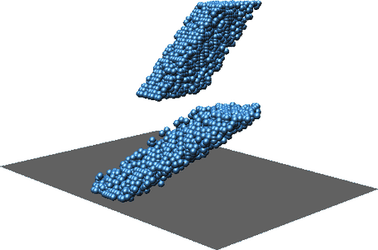Figure: One scatter plot of two variables (left), the same scatter plot but with color showing third variable (middle), again the same scatter plot in 3D where Z represents a third variable (right).Figure: One scatter plot in with one variable as Z coordinate and another variable as color (two rotated views).

### Layout

When working only with variable, X axis represents the first one and Y axis the second one. With more than one variable, the individual scatter plots for individual pairs of variables are at the same place. In this case, the coordinates show the actual values of the variables. Each scatter plot is placed into a separate layer of the output vector map.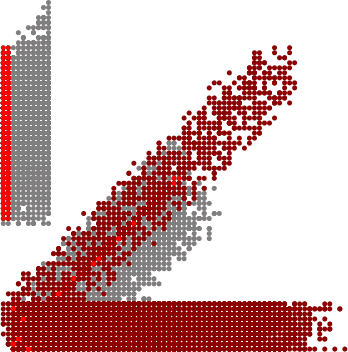Figure: Three overlapping scatter plots of three variables A, B, and C. Individual scatter plots are distinguished by color. The colors can be obtained using d.vect layer=-1 -c.

If visualization is more important than preserving the actual values, the -s flag can be used. This will place the scatter plots next to each other separated by values provided using spacing option.

The layout options can be still combined with additional variables represented as Z coordinate or color. In that case, Z coordinate or color is same for all the scatter plots.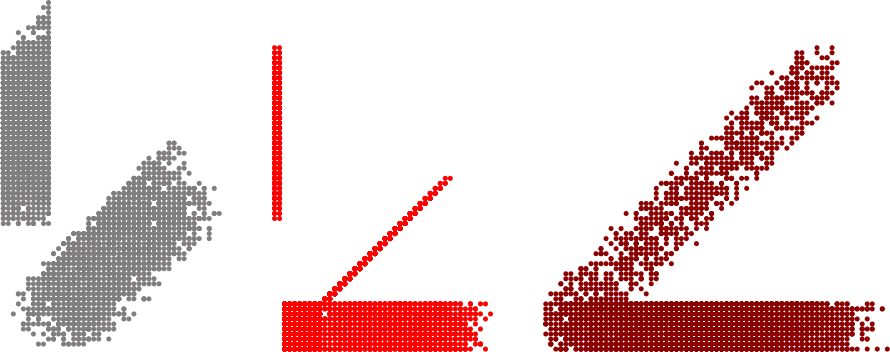Figure: Three scatter plots of three variables A, B, and C. First one is A and B, second A and C, and third B and C.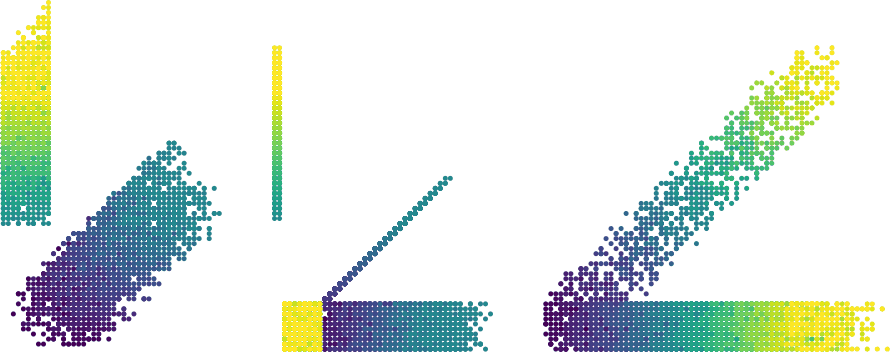Figure: Three scatter plots of three variables A, B, and C with color showing a fourth variable D in all scatter plots.

The options xscale, yscale and zscale will cause the values to be rescaled before they are stored as point coordinates. This is useful for visualization when one of the variables has significantly different range than the other or when the scatter plot is shown with other data and must fit a certain area. The position option is used to place the scatter plot to any given coordinates. Similarly, -w flag can be used to place it to the south-west corner of the computation region.

## NOTES

The resulting vector will have as many points as there is 3D raster cells in the current computational region. It might be appropriate to use coarser resolution for the scatter plot than for the other computations. However, note that the some values will be skipped which may lead, e.g. to missing some outliers.

The color_raster input is expected to be categorical raster or have values which won't loose anything when converted from floating point to integer. This is because vector categories are used to store the color_raster values and carry association with the color.

The visualization of the output vector map has potentially the same issue as visualization of any vector with many points. The points cover each other and above certain density of points, it is not possible to compare relative density in the scatter plot. Furthermore, if colors are associated with the points, the colors of points rendered last are those which are visible, not actually showing the prevailing color (value). The modules v.mkgrid and v.vect.stats can be used to overcome this issue.

## EXAMPLES

### Example with generated data

Set the computational region for generating data and subsequent analysis:
```g.region s=0 n=100 w=0 e=100 b=0 t=50 res=10 res3=10 tbres=1 -p3
```
Generate data using raster algebra:
```r3.mapcalc "A = if(depth() < ndepths()/ 2,  row()+col()+depth(), col())" -s
r3.mapcalc "B = if(row() < nrows()/2, rand(0, nrows()) + col() + depth(), depth() + rand(0, nrows()))" -s
r3.mapcalc "C = if(col() < ncols()/3, row() + depth(), rand(0, ncols()))" -s
r3.mapcalc "D = depth()"
```
Create a scatter plot of correlations between A and B. Use C for the Z axes (height) and D for the color.
```r3.scatterplot input=A,B output=scatterplot z_raster=C color_raster=D
```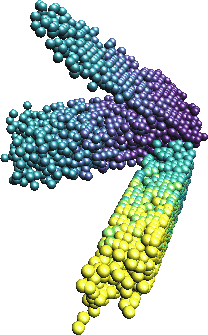Figure: 2D scatter plot showing third variable as color and 3D scatter plot showing third variable as color and fourth variable on Z axis which uncovers another group of values not visible from the 2D scatter plot

r3.stats, d.correlate, v.mkgrid, v.vect.stats, g.region

## AUTHOR

Vaclav Petras, NCSU GeoForAll Lab

## SOURCE CODE

Available at: r3.scatterplot source code (history)

Latest change: Mon Jun 28 07:54:09 2021 in commit: 1cfc0af029a35a5d6c7dae5ca7204d0eb85dbc55

Note: This addon document is for an older version of GRASS GIS that will be discontinued soon. You should upgrade your GRASS GIS installation, and read the current addon manual page.

© 2003-2023 GRASS Development Team, GRASS GIS 7.8.8dev Reference Manual